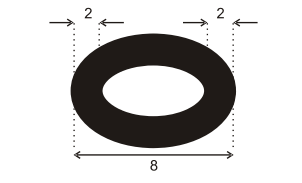# IMAT 2011 Q39 [Chain]

A chain link has the following dimensions:If you join six of these links together, and stretch the chain to its full extent, what is the total length of the chain?

A. 24
B. 28
C. 30
D. 32
E. 36

Some Quick Questions to ask yourself when doing this type of problem solving:

• What is being asked? Underline the specifics in the question.
• Analyse the data, is there anything we can eliminate immediately?
• What information do I need to solve the question? Where can I find it?
• How can I draw or use the graph to help me?

For this question I would recommend drawing a picture. Here is a sample picture. Although it seems chaotic, this is something you can sketch quickly and effectively. This image breaks down every link into 2 unit sections so we have a common number. Now that we have this, we just need to realize that the middle sections are shorter because they have an overlap on both sides and the end pieces are longer.

Adding up our results from the image:

2(14)=28

How could we do a more mathematical approach?

We know that when there is an overlap, the link will lose 2 units from the inside. We can formulate the expression 4(8-4) for the inner pieces because there is 4 links each with connections on both sides. The end units are longer as they only missing 2 units from one side, so the expression can be 2(8-4).

2(8-2)+4(8-4)=28